# unknown solutions A and B are 0.1 M solutions of either HCI or NaOH. According to your pH measurement, which is which...

unknown solutions A and B are 0.1 M solutions of either HCI or NaOH. According to your pH measurement, which is which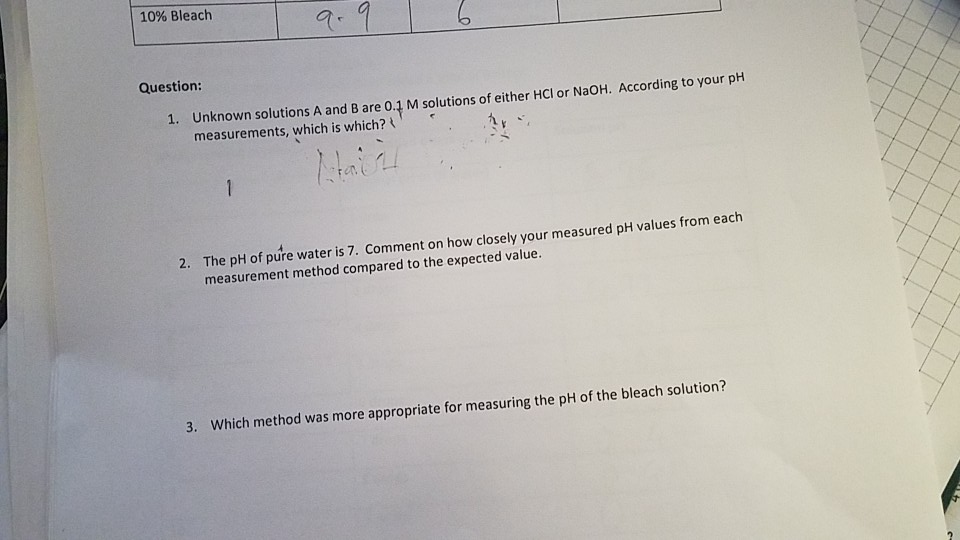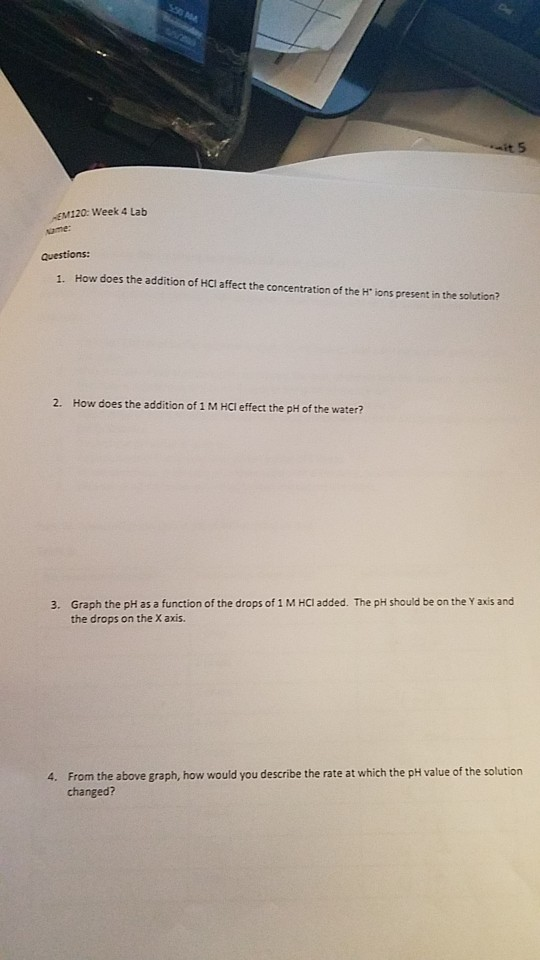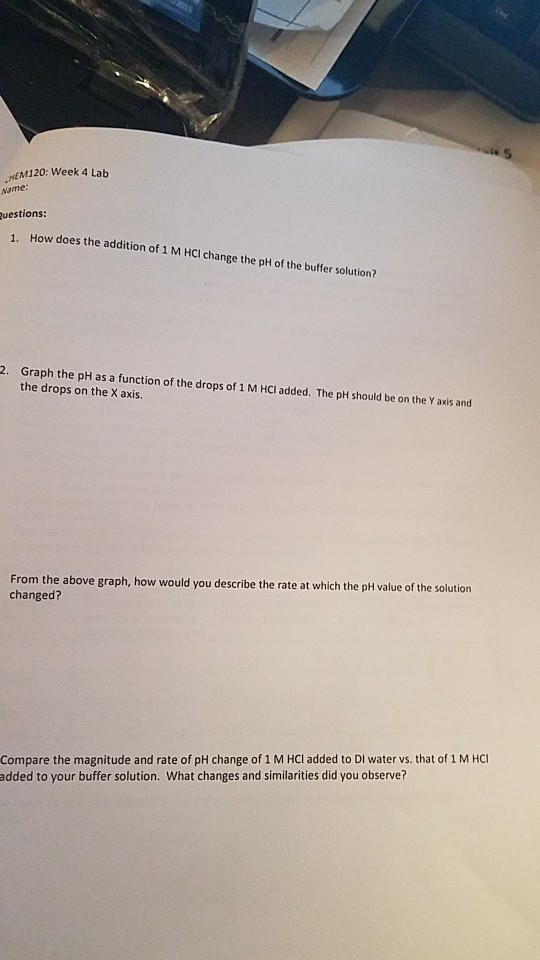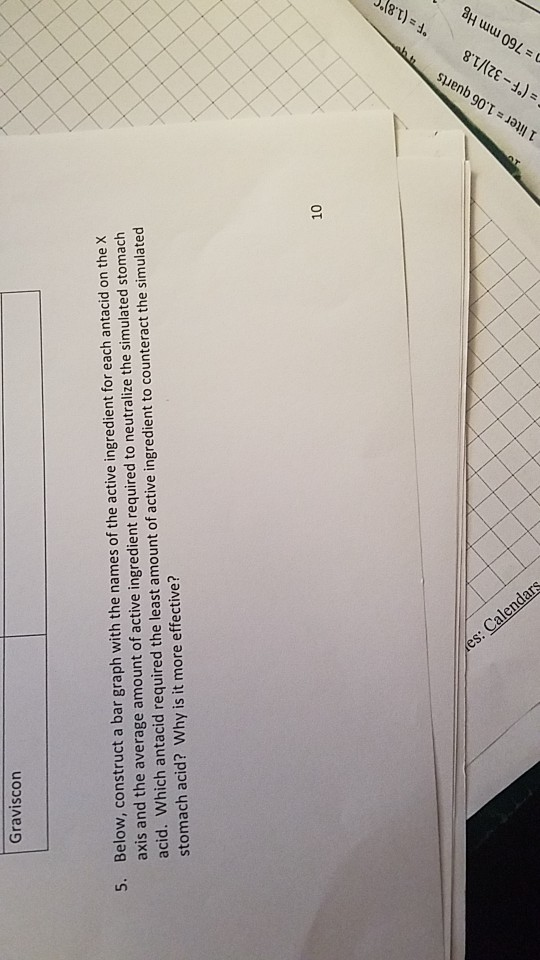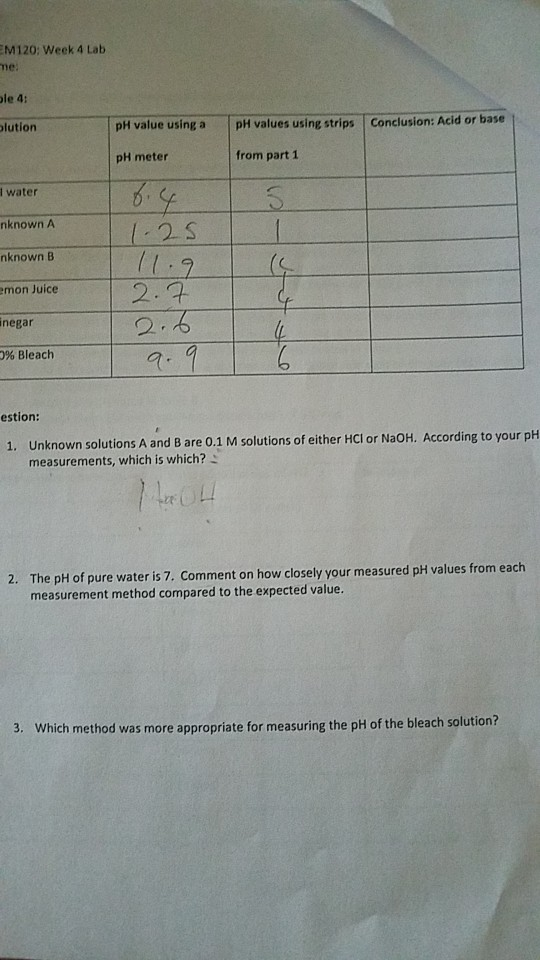10% Bleach Question: 1. Unknown solutions A and B are 0.1 M solutions of either HCl or NaOH. According to your pH measurements, which is which? 1 2. The pH of pure water is 7. Comment on how closely your measured pH values from each measurement method compared to the expected value. Which method was more appropriate for measuring the pH of the bleach solution? 3.
it 5 -EM120: Week 4 Lab Aame: Questions: 1. How does the addition of HCl affect the concentration of the H" ions present in the solution? 2. How does the addition of 1 M HCl effect the pH of the water? 3. Graph the pH as a function of the drops of 1 M HCl added. The pH should be on the Y axi the drops on the X axis. 4. From the above graph, how would you describe the rate at which the pH value of the solution changed?
6019 HEM120: Week 4 Lab Name: Questions: How does the addition of 1 M HCl change the pH of the buffer solution? 1 2. Graph the pH as a function of the drops of 1M HCI added. The pH should be on the Y axis and the drops on the X axis. From the above graph, how would you describe the rate at which the pH value of the solution changed? Compare the magnitude and rate of pH change of 1 M HCI added to DI water vs. that of 1 M HCl added to your buffer solution. What changes and similarities did you observe?
es: CalendarS F= (1.8) 8T) .06 90 qua (F-32) / 1.8 . =. = 760 mm Hg er 1 -.) Graviscon Below, construct a bar graph with the names of the active ingredient for each antacid on the X axis and the average amount of active ingredient required to neutralize the simulated stomach acid. Which antacid required the least amount of active ingredient to counteract the simulated stomach acid? Why is it more effective? 5. OT
EM120: Week 4 Lab me: le 4: olution pH value using a pH values using strips Conclusion: Acid or base from part 1 pH meter I water nknown A 125 nknown B 2.7 2.6 emon Juice negar 0% Bleach estion: 1. Unknown solutions A and B are 0.1M solutions of either HCI or NaOH. According to your pH measurements, which is which? 7 L04 The pH of pure water is 7. Comment on how closely your measured pH values from each measurement method compared to the expected value. 2. 3. Which method was more appropriate for measuring the pH of the bleach solution?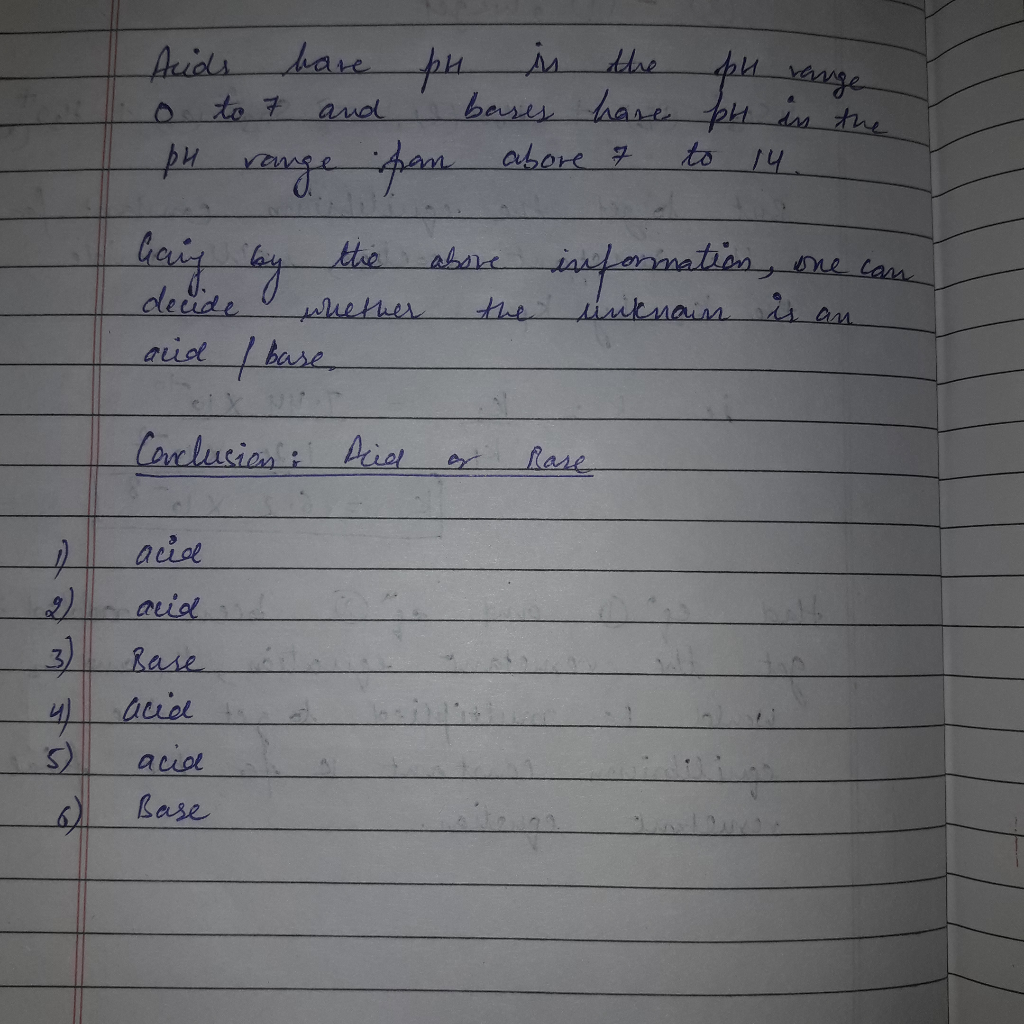#### Earn Coin

Coins can be redeemed for fabulous gifts.

Similar Homework Help Questions
• ### unknown solutions A and B are 0.1 M solutions of either HCI or NaOH. According to...unknown solutions A and B are 0.1 M solutions of either HCI or NaOH. According to your pH measurement, which is which 10% Bleach Question: 1. Unknown solutions A and B are 0.1 M solutions of either HCl or NaOH. According to your pH measurements, which is which? 1 2. The pH of pure water is 7. Comment on how closely your measured pH values from each measurement method compared to the expected value. Which method was more appropriate for...

• ### In this experiment you will be measuring pH for several different solutions. A pH probe will...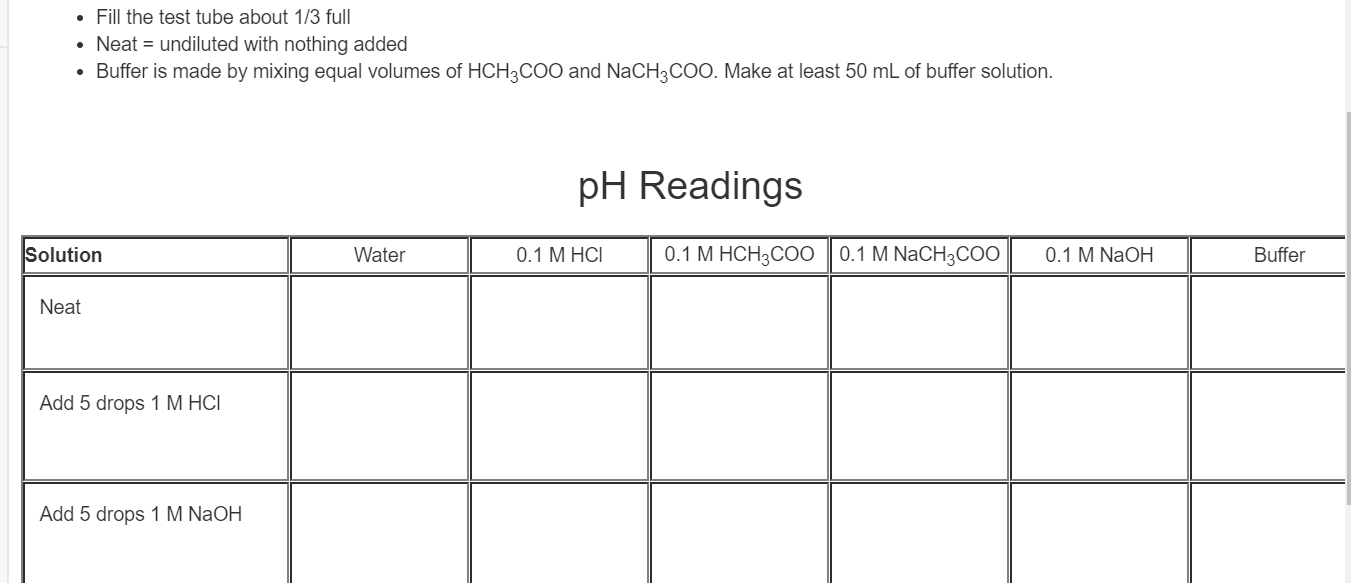In this experiment you will be measuring pH for several different solutions. A pH probe will be used rather than pH paper as it will be more accurate. You will be testing the pH of six solutions: deionized water, 0.1 M HCl, 0.1 M HCH3COO, 0.1 M NaCH3COO, 0.1 M NaOH, and a buffer made by mixing equal parts of HCH3COO and NaCH3COO. You will measure the pH of each solution three times: Neat - just the solution After the...

• ### REPOSTING: observing pH changes in Water and Buffer solutions. the amount added of HCl and NaOH...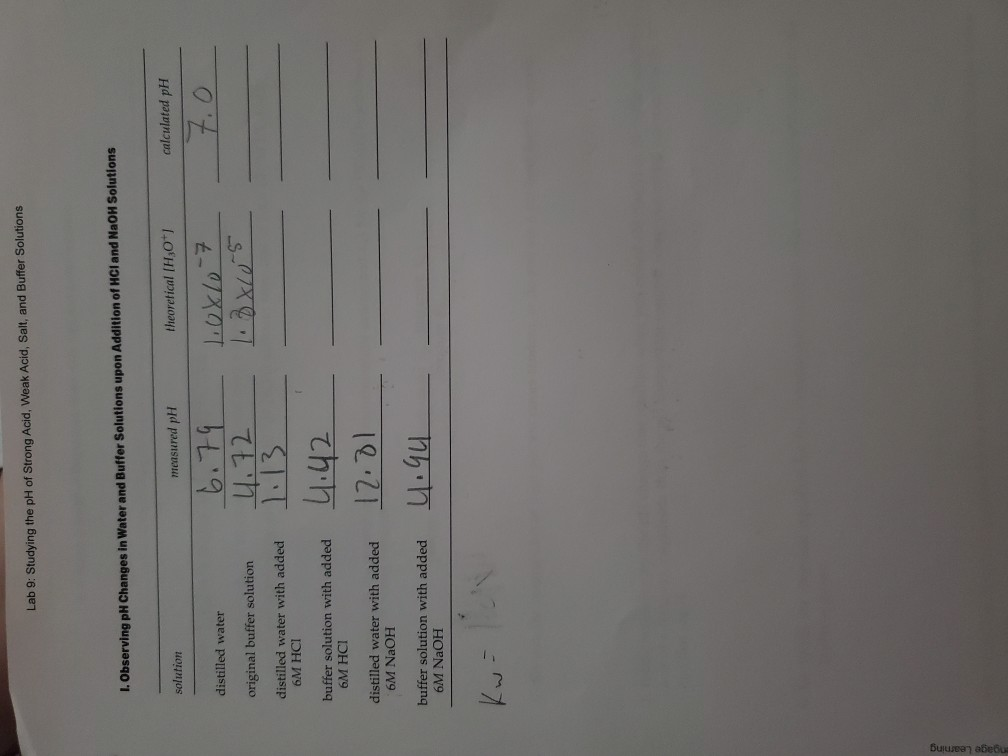REPOSTING: observing pH changes in Water and Buffer solutions. the amount added of HCl and NaOH are both 25mL. the buffer solution is made of 2grams of NaC2H3O2 and 4 mL of 6M HC2H3O2 in a 50 mL solution (46mL of water). the solution will contain 2.4x10^-2 mol each of NaC2H3O2 a d HC2H3O2. question: how to answer it (I'm not sure I'm doing it right and want an expert to double check): I need help calculating the theoretical and...

• ### considering data from part D which solution was the most effective at resisting a change in ph when NaOH was added? off...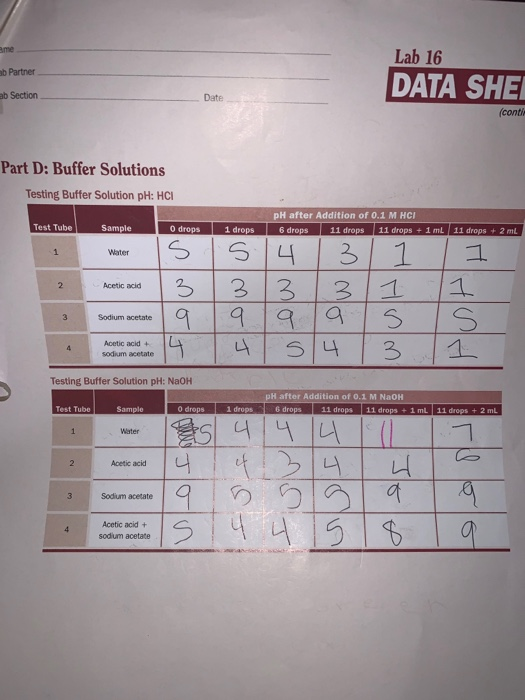considering data from part D which solution was the most effective at resisting a change in ph when NaOH was added? offer an explanation for this difference in behavior compared to the other solutions. ame Lab 16 ab Partner DATA SHE ab Section Date (conti Part D: Buffer Solutions Testing Buffer Solution pH: HC pH after Addition of 0.1 M HCI Test Tube 0 drops Sample 1 drops 6 drops 11 drops 11 drops + 1 mL 11 drops +2...

• ### Calcium carbonate, CaCO3, reacts with stomach acid, (HCI, hydrochloric acid) according to the following equation: CaCO3(s)...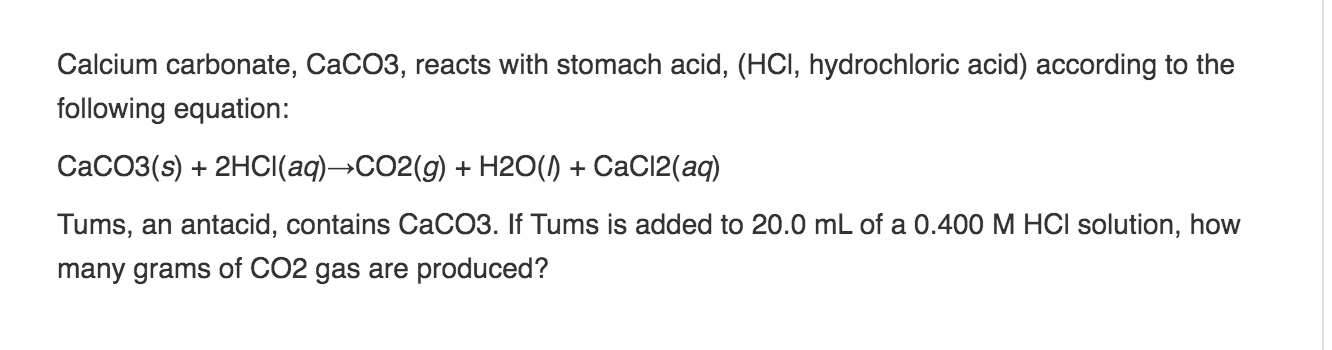Calcium carbonate, CaCO3, reacts with stomach acid, (HCI, hydrochloric acid) according to the following equation: CaCO3(s) + 2HCl(aq)-CO2(g) + H2O(1) +CaCl2(aq) Tums, an antacid, contains CaCO3. If Tums is added to 20.0 mL of a 0.400 M HCl solution, how many grams of CO2 gas are produced?

• ### Which of the following solutions is the most basic ? 0.1 M HCI a solution PH=9.5...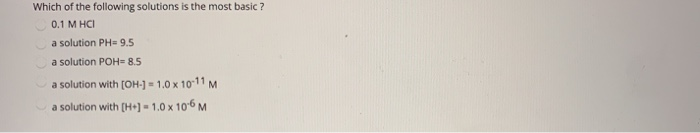Which of the following solutions is the most basic ? 0.1 M HCI a solution PH=9.5 a solution POH=8.5 a solution with [OH-] = 1.0 x 10-11 M a solution with (H+) -1.0 x 10-6 M Calculate the equilibrium constant (K) for a balanced redox reaction that involves 6 electrons and has AG= 410000 joules at 25°C. a. 1.4 * 1072 b.8.9 x 10-73 C.1.35 x 10-72 d. 1,5x1024 0.4.6 x 10 31

• ### \    Part 3: Preparing and testing the carbonic acid/bicarbonate buffer 3) and 6) Buffer 0.1...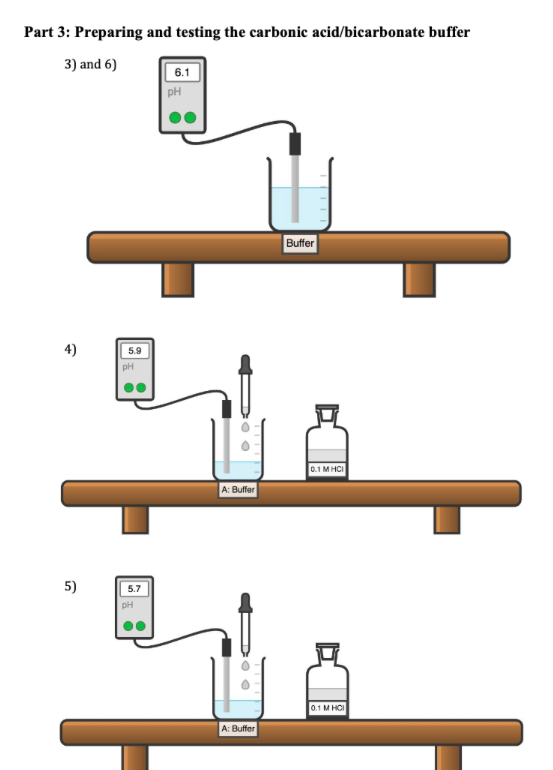\    Part 3: Preparing and testing the carbonic acid/bicarbonate buffer 3) and 6) Buffer 0.1 MHCI A: Buffer A: Buffer B: Butter B: Buffer Part 4: Testing water for pH resistance 2) and 5) DFDFDF A: HO B: H0 Question 6 1 pts pH in Beaker A pH in Beaker B After 5 drops NaOH Solution Initial After 5 drops HCI After 20 drops HCI Initial After 20 drops NaOH Buffer (part 3) Water (part 4) Use the data...

• ### 3. How many moles of HCl are there in 0.1 L of 0.1 M HCI? 4....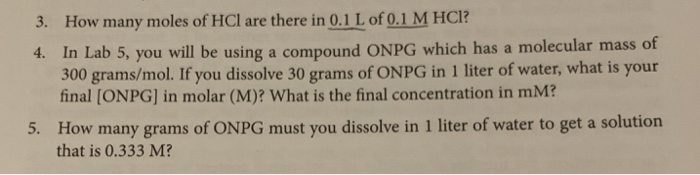3. How many moles of HCl are there in 0.1 L of 0.1 M HCI? 4. In Lab 5, you will be using a compound ONPG which has a molecular mass of 300 grams/mol. If you dissolve 30 grams of ONPG in 1 liter of water, what is your final (ONPG] in molar (M)? What is the final concentration in mM? 5. How many grams of ONPG must you dissolve in 1 liter of water to get a solution that...

• ### 174 Problems and questions 1. Compare and contrast the three methods used to test pH (red...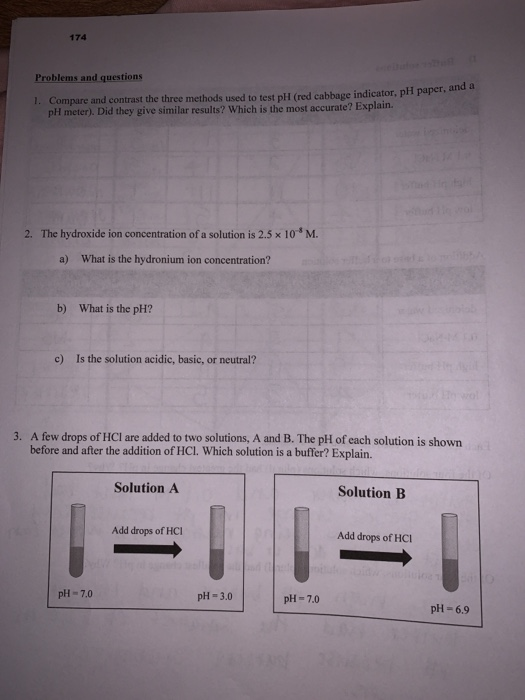174 Problems and questions 1. Compare and contrast the three methods used to test pH (red cabbage indicator, pH paper, and a pH meter). Did they give similar results? Which is the most accurate? Explain. 2. The hydroxide ion concentration of a solution is 2.5 x 10 M. What is the hydronium ion concentration? a) What is the pH? b) Is the solution acidic, basic, or neutral? c) 3. A few drops of HCl are added to two solutions, A...

• ### Post Lab Activity Acids, Bases, pH, and Buffers 1. Calculate the pH of a 0.08 M NaOH solution. 2. Hydrochloric acid...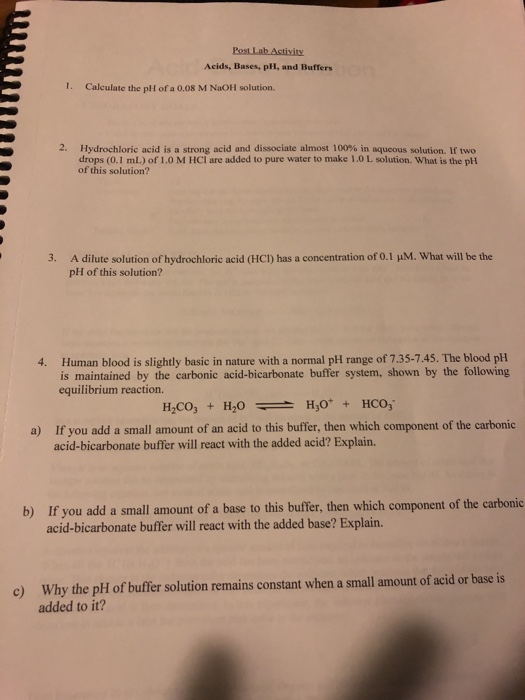Post Lab Activity Acids, Bases, pH, and Buffers 1. Calculate the pH of a 0.08 M NaOH solution. 2. Hydrochloric acid is a strong acid and dissociate almost 100% in aqueous solution. If two drops (0.1 mL) of 1.0 MHCI are added to pure water to make 1.0 L solution. What is the pH of this solution? 3. M. What will be the A dilute solution of hydrochloric acid (HCI) has a concentration of 0.1 pH of this solution? 4....